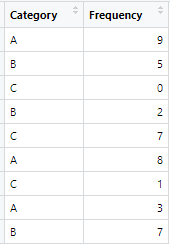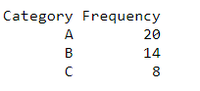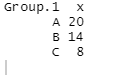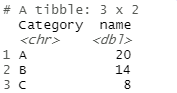# How to sum a variable by group in R?

• Last Updated : 01 Apr, 2021

In this article, let’s discusses how to find sum of a variable by the group in R Programming Language.

Dataset in Use:Table-1

Expected output:Group by Category

### Approach

• Create dataframe
• Set values to required parameters
• Pass to the function in use
• Display dataframe

### Method 1: Using aggregate function

In this method we will take help of aggregate function to sum by group. Aggregate function splits the data into subsets, computes summary statistics for each, and returns the result in a convenient form.

Syntax:

aggregate(x = dataset_Name , by = group_list, FUN = any_function)  # Basic R syntax of aggregate function

Example:

## R

 `GFG <- ``data.frame``(``   ``Category  = ``c ``(``"A"``,``"B"``,``"C"``,``"B"``,``"C"``,``"A"``,``"C"``,``"A"``,``"B"``), ``   ``Frequency= ``c``(9,5,0,2,7,8,1,3,7)``)`` ` `aggregate``(x= GFG\$Frequency,              ``         ``by = ``list``(GFG\$Category),        ``         ``FUN = sum)                      `

Output:### Method 2: Using dplyr

dplyr is a package which provides a set of tools for efficiently manipulating datasets in R

Methods in dplyr package

• mutate() adds new variables that are functions of existing variables
• select() picks variables based on their names.
• filter() picks cases based on their values.
• summarise() reduces multiple values down to a single summary.
• arrange() changes the ordering of the rows.

Before using this package you have to install it

Program:

## R

 `library``(``"dplyr"``)                             ``GFG <- ``data.frame``(``   ``Category  = ``c ``(``"A"``,``"B"``,``"C"``,``"B"``,``"C"``,``"A"``,``"C"``,``"A"``,``"B"``), ``   ``Frequency= ``c``(9,5,0,2,7,8,1,3,7)``)``GFG%>%                                         `` ``group_by``(Category) %>%                        `` ``summarise_at``(``vars``(Frequency),                 ``              ``list``(name = sum))`

Output:My Personal Notes arrow_drop_up